# A Guide to Third-Person Controllers

You can access the full course here: Develop a 3D Action RPG with Godot

### Player Setup

In this lesson, we are going to begin to create our player.

#### Creating Player Scene

Click on Scene > New Scene.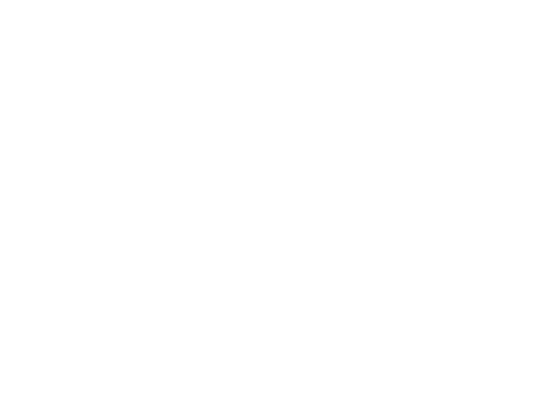Click on Other Nodes in the Scene window.
Search for ‘KinematicBody‘ in the Create New Node window.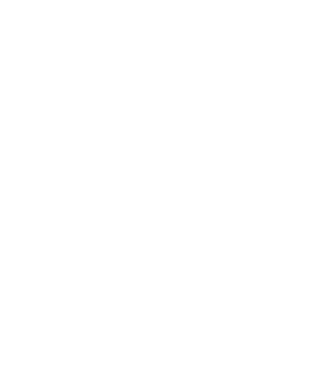Select the ‘KinematicBody‘ node and hit ‘Create‘.
The new node is now visible in the Scene window. Rename it to “Player”.
Save the scene as “Player.tscn”.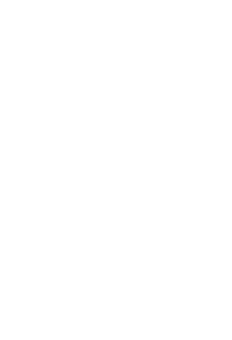#### Creating Components (1) – Mesh

Right-click on the player node and select ‘Add a child’ (Ctrl+A)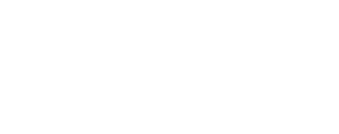Create a new MeshInstance.
Click on ‘Mesh‘ and select ‘New CapsuleMesh‘ in the Inspector.
Set the radius to be 0.5, the y-translation to be 1, and the x-rotation to be 90.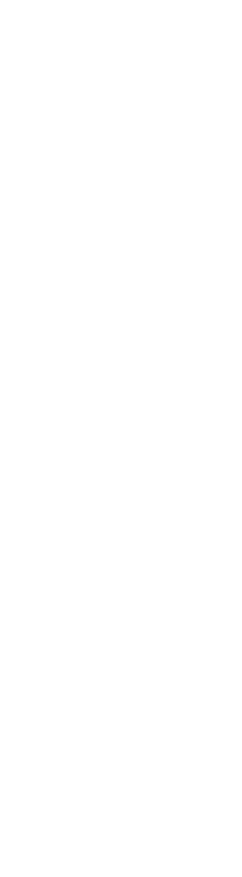#### Creating Components (2) – CollisionShape

Create another child of type CollisionShape.
Set the Shape to be ‘Capsule’, the radius to be 0.5, the y-translation to be 1, and the x-rotation to be 90, so it matches up with our capsule mesh.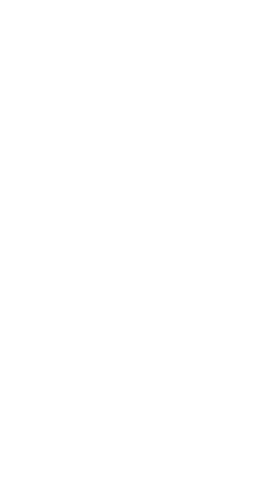#### Creating Components (3) – Camera

Create a child node of the player, which is of type Camera.
Create another child of type Spatial and rename it to “CameraOrbit”. Drag in the camera as a child.
Set the CameraOrbit‘s position to be (0, 1, 0) so it can be orbiting around the center of the player.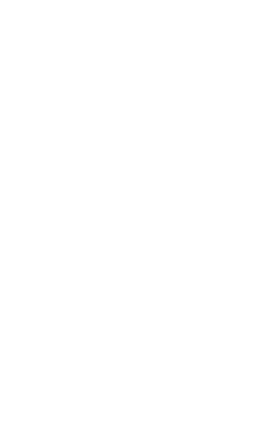Set the camera position to be (-1, 1, -5) and the rotation to be (0, 180, 0). Click on the ‘Preview‘ to see our default camera view in game.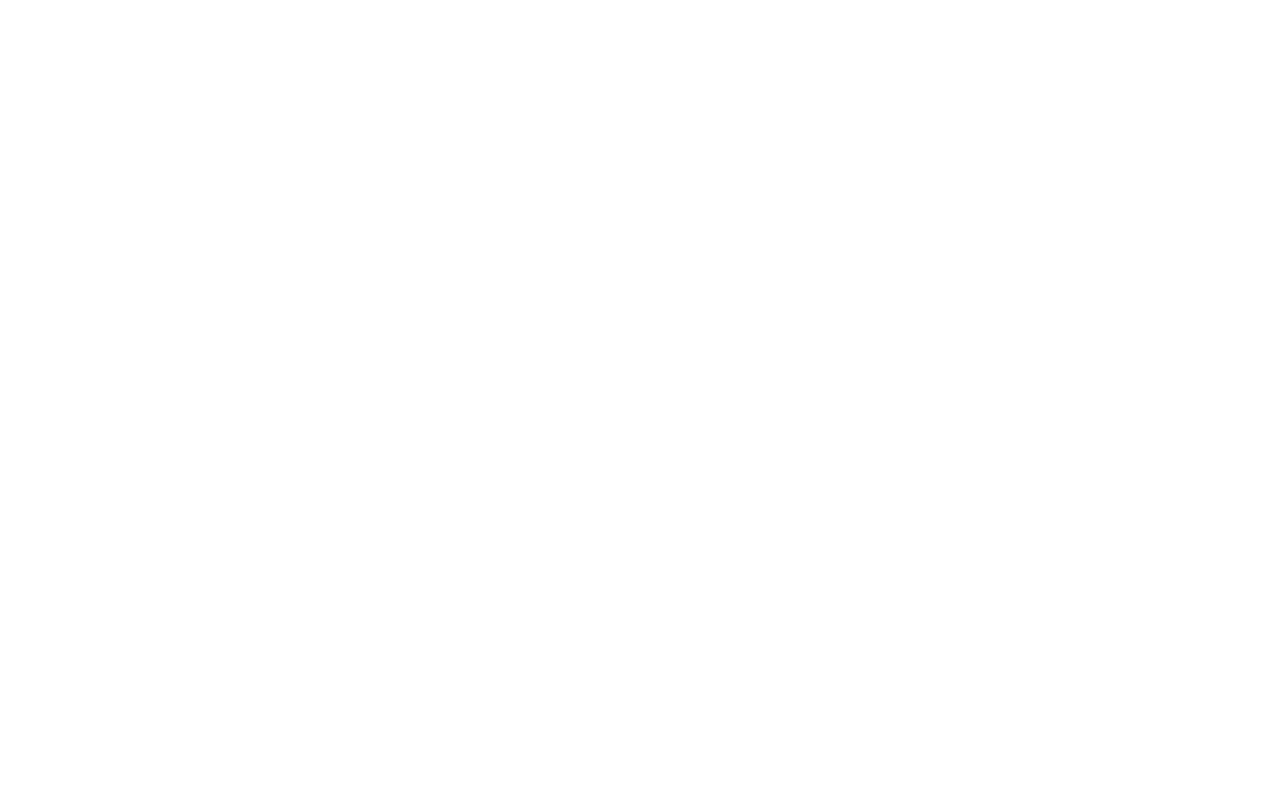#### Creating Components (4) – Weapon Holder

Create a new Spatial node and rename it to be “WeaponHolder”. Set the position to be (-0.58, 1, 0.035)
Drag in the sword model file (Models > Sword.dae) as a child of WeaponHolder. Set the position to be (0, 0, 0), the size to be (0.15, 0.15, 0.15) and the rotation to be (0, 90, 45).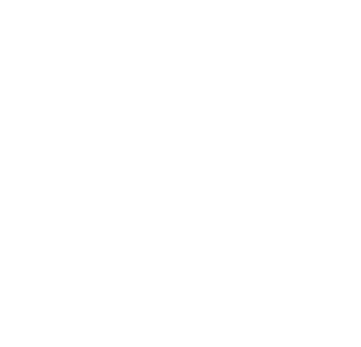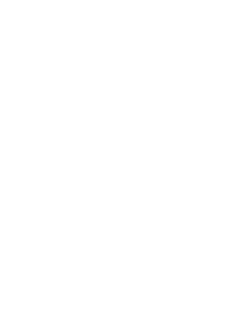#### Creating Components (5) – Attack Ray Cast

Create a node of type RayCast. This is going to be how we detect if we’re looking at an enemy when we attack them.
Set the properties as below, so it is in the middle of the player but a little bit forward of the sword.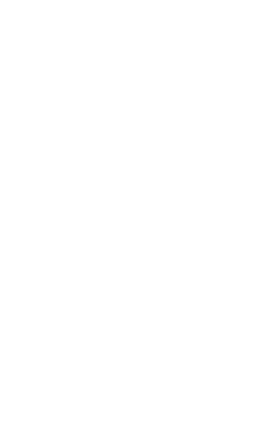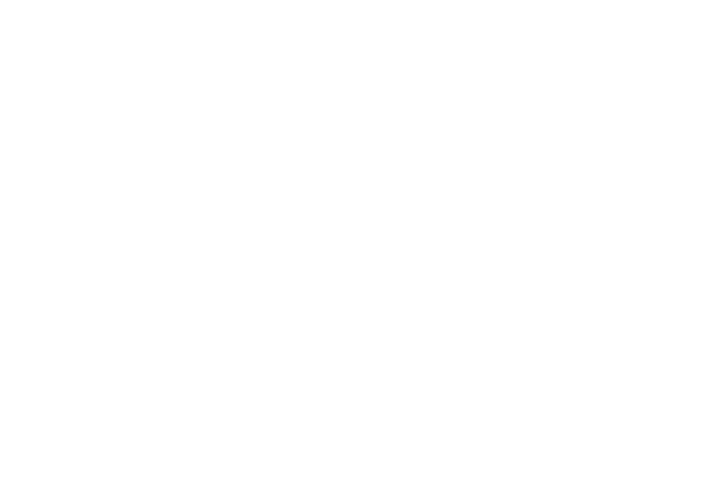Finally, drag in the player scene (Player.tscn) into the main scene and set the position to be (0, 0, 0) so they’re in the middle of the level.
### Camera Setup

In this lesson, we’re going to set up a camera look.

Click on the CameraOrbit node and create a new script.
Save the script as “CameraOrbit.gd”.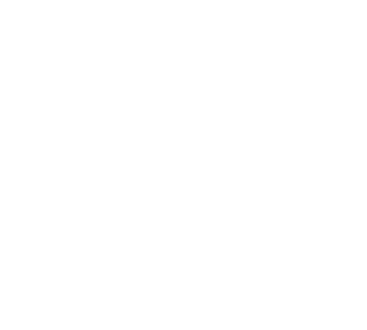In the script, we’ll create a float variable called lookSensitivity, and set its default value to be 15.0.

```extends Spatial

var lookSensitivity : float = 15.0```

This is going to be based on how fast our mouse moves.

We’ll then set up the min/max angles for the vertical rotation of the camera.

```extends Spatial

var lookSensitivity : float = 15.0
var minLookAngle : float = -20.0
var maxLookAngle : float = 75.0```

Now we need to keep track of the mouse delta, which tells how fast and in what direction did the mouse move for each frame. This is going to be Vector2 for an X and Y in screen coordinates.
```extends Spatial

var lookSensitivity : float = 15.0
var minLookAngle : float = -20.0
var maxLookAngle : float = 75.0

var mouseDelta : Vector2 = Vector2()```

Lastly, we need to get access to our parent (player) node. Note that the onready keyword is used to ensure that the node is found as soon as the game starts.
```extends Spatial

var lookSensitivity : float = 15.0
var minLookAngle : float = -20.0
var maxLookAngle : float = 75.0

var mouseDelta : Vector2 = Vector2()

onready var player = get_parent()```

Now, we’re going to use a built-in function called _input(event). The event parameter contains all the information of whatever’s being pressed on your input devices (e.g. keyboard).
`func _input(event):`

Using this event parameter, we can get how fast and in what direction the mouse has moved each frame. We’ll store this into our mouseDelta variable.
```func _input(event):

if event is InputEventMouseMotion:
mouseDelta = event.relative```

We can then create another built-in function called _process(delta), which will be called 60 times a second.
`func _process(delta):`

The delta parameter is the duration between each frame, and we can use this to do stuff based on ‘per second’ rather than ‘per frame’.

Now we want to get the rotation– what rotation are we going to be applying to the camera / our player? This will be stored in a variable called “rot” as a Vector3 (X,Y,Z).

```func _process(delta):

var rot = Vector3(mouseDelta.y, mouseDelta.x, 0) * lookSensitivity * delta```

We’ll assign mouseDelta.y for the x, because if we’re moving along the x-axis, it will be vertical movement (up and down). For the same reason, we’ll apply MouseDelta.x for the y, and zero for the z.

Now we need to apply this rotation to the camera and to the player. Let’s apply the vertical rotation first (x -axis)

```func _process(delta):

var rot = Vector3(mouseDelta.y, mouseDelta.x, 0) * lookSensitivity * delta

rotation_degrees.x += rot.x```

Currently, if we keep moving our mouse up, it’s going to eventually do a loop around the player. Hence, we’ll clamp the position:
```func _process(delta):

var rot = Vector3(mouseDelta.y, mouseDelta.x, 0) * lookSensitivity * delta

rotation_degrees.x += rot.x
rotation_degrees.x = clamp(rotation_degrees.x, minLookAngle, maxLookAngle)```

Now we need to rotate it sideways, but this time we’re not going to be rotating the camera, we’re going to rotate the entire player.
```func _process(delta):

var rot = Vector3(mouseDelta.y, mouseDelta.x, 0) * lookSensitivity * delta

rotation_degrees.x += rot.x
rotation_degrees.x = clamp(rotation_degrees.x, minLookAngle, maxLookAngle)

player.rotation_degrees.y -= rot.y```

Finally, we’ll clear our mouse delta so we won’t keep rotating around in the same direction if we stop our mouse.
```func _process(delta):

var rot = Vector3(mouseDelta.y, mouseDelta.x, 0) * lookSensitivity * delta

rotation_degrees.x += rot.x
rotation_degrees.x = clamp(rotation_degrees.x, minLookAngle, maxLookAngle)

player.rotation_degrees.y -= rot.y

mouseDelta = Vector2()```

At the start of the game, the mouse cursor should be captured in the center, so our mouse doesn’t fall off the screen. This can be done in the _ready() function, which is called once when the node is initialized.
```func _ready():

Input.set_mouse_mode(Input.MOUSE_MODE_CAPTURED)```

```extends Spatial

var lookSensitivity : float = 15.0
var minLookAngle : float = -20.0
var maxLookAngle : float = 75.0

var mouseDelta : Vector2 = Vector2()

onready var player = get_parent()

Input.set_mouse_mode(Input.MOUSE_MODE_CAPTURED)

func _input(event):

if event is InputEventMouseMotion:
mouseDelta = event.relative

func _process(delta):

var rot = Vector3(mouseDelta.y, mouseDelta.x, 0) * lookSensitivity * delta

rotation_degrees.x += rot.x
rotation_degrees.x = clamp(rotation_degrees.x, minLookAngle, maxLookAngle)

player.rotation_degrees.y -= rot.y

mouseDelta = Vector2()```

We can now rotate our camera around the player as much as we want and our mouse won’t fall off the screen. (Press Alt+F4 to quit the game window)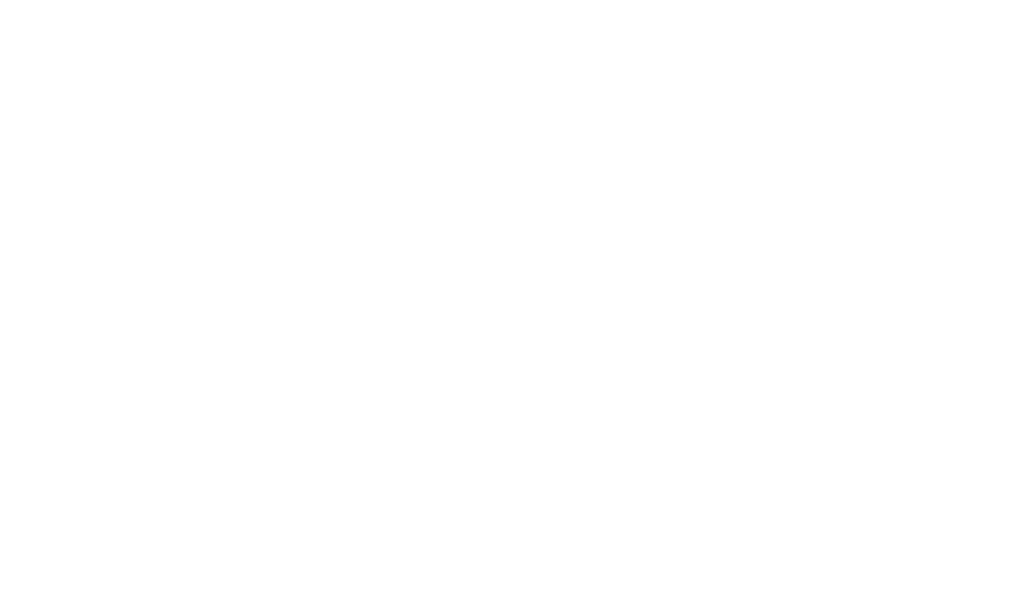### Inputs & Player Script

In this lesson, we’re going to begin to script our player.

#### Setting Up Input Keys

Go to Project > Project Settings…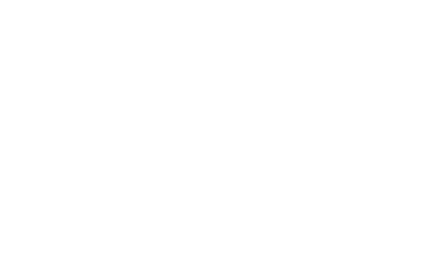Open up the ‘Input Map‘ tab in the project settings.
In this window, we can create new actions by giving the action names.

• ‘move_forward’
• ‘move_backward’
• ‘move_right’
• ‘move_left’
• ‘jump’
• ‘attack’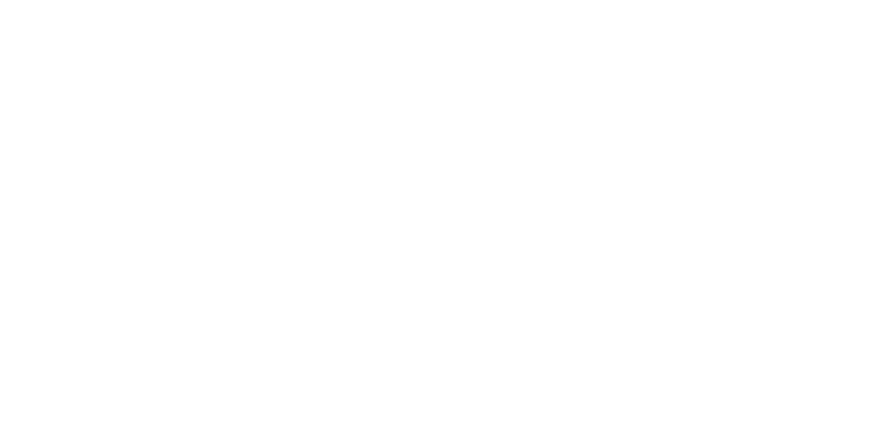We can then assign a unique key for each action by clicking on the ‘+’ button and pressing the relevant key on your keyboard.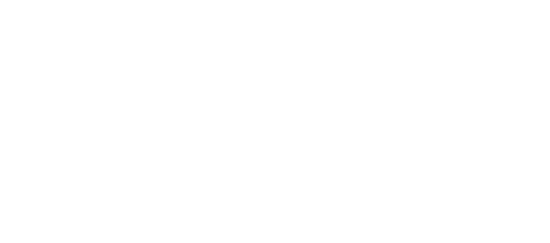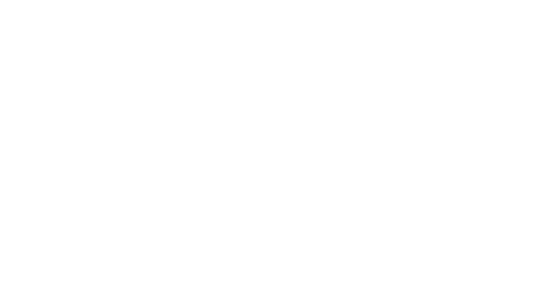We’ll move with W/A/S/D, jump with Space, and attack with the LMB.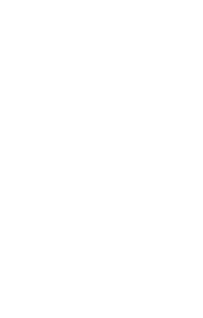Once that’s all set up, close the project settings window.

#### Creating a new script

With the Player node selected, if you go to the Inspector panel, you will see that there is a Script tab. Expand it and click on [empty]. Then select ‘New Script’ in the dropdown.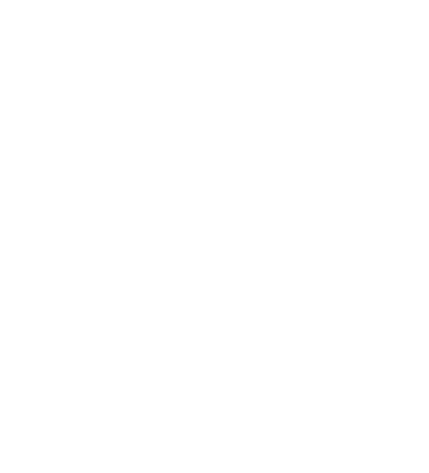Hit on the ‘Create’ button.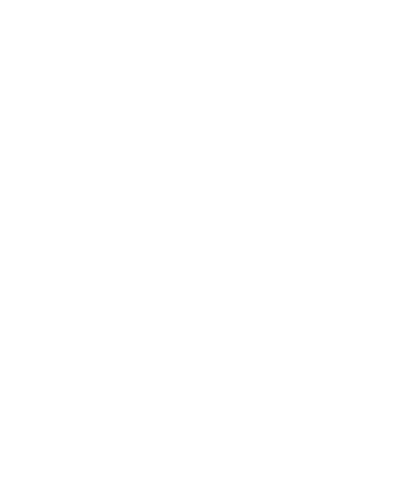This will automatically open up a Scripting Interface.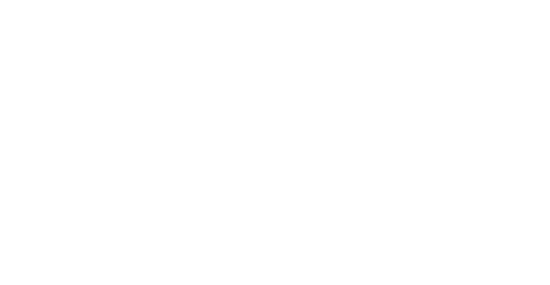#### Scripting The Player

First of all, we’re going to create variables for our game– starting with the basic stats.

```extends KinematicBody

var curHp : int = 10
var maxHp : int = 10
var damage : int = 1```

Later on, we’re going to implement gold so we can collect coins.
```extends KinematicBody

var curHp : int = 10
var maxHp : int = 10
var damage : int = 1

var gold : int = 0```

We also need to know for attacking, how often can we attack? What’s the minimum amount of time between clicks?
```extends KinematicBody

var curHp : int = 10
var maxHp : int = 10
var damage : int = 1

var gold : int = 0

var attackRate : float = 0.3
var lastAttackTime : int = 0```

We need to create variables for physics stuffs, such as moving and jumping.
```extends KinematicBody

var curHp : int = 10
var maxHp : int = 10
var damage : int = 1

var gold : int = 0

var attackRate : float = 0.3
var lastAttackTime : int = 0

var moveSpeed : float = 5.0
var jumpForce : float = 10.0
var gravity : float = 15.0

var vel : Vector3 = Vector3()```

Finally, we’re going to get access to our Camera and Raycast.
```extends KinematicBody

var curHp : int = 10
var maxHp : int = 10
var damage : int = 1

var gold : int = 0

var attackRate : float = 0.3
var lastAttackTime : int = 0

var moveSpeed : float = 5.0
var jumpForce : float = 10.0
var gravity : float = 15.0

var vel : Vector3 = Vector3()

onready var camera = get_node("CameraOrbit")
onready var attackRayCast = get_node("AttackRayCast")```

Now, we’ll reset our velocity inside physics_process(), which gets called 60 times a second. This is so that we can stop immediately when we’re not pressing a button.
```func _physics_process(delta):

vel.x = 0
vel.z = 0```

Now we’ll store our input in a vector3 component whenever we press on a button. If an action is pressed, we need to modify this input vector accordingly.
```func _physics_process(delta):

vel.x = 0
vel.z = 0

var input = Vector3()

if Input.is_action_pressed("move_forward"):
input.z += 1
if Input.is_action_pressed("move_backward"):
input.z -= 1
if Input.is_action_pressed("move_left"):
input.x += 1
if Input.is_action_pressed("move_right"):
input.x -= 1```

The magnitude of this vector is all the three axes (X,Y,Z) added together. So if we’re moving forward, the magnitude is going to be 1.

If we’re moving forward and left, the magnitude of that is going to be 2. This will result in moving twice the speed if we move diagonally. We can prevent this by normalizing the vector.

```func _physics_process(delta):

vel.x = 0
vel.z = 0

var input = Vector3()

if Input.is_action_pressed("move_forward"):
input.z += 1
if Input.is_action_pressed("move_backward"):
input.z -= 1
if Input.is_action_pressed("move_left"):
input.x += 1
if Input.is_action_pressed("move_right"):
input.x -= 1

input = input.normalized()```

Now we need to figure out which direction we are facing because when we press left or right, we want to move based on our local direction (instead of the global direction).

This can be done by multiplying our forward direction (transform.basis.z) by our z input, plus our right direction (transform.basis.x) by our x input.

```func _physics_process(delta):

vel.x = 0
vel.z = 0

var input = Vector3()

if Input.is_action_pressed("move_forward"):
input.z += 1
if Input.is_action_pressed("move_backward"):
input.z -= 1
if Input.is_action_pressed("move_left"):
input.x += 1
if Input.is_action_pressed("move_right"):
input.x -= 1

input = input.normalized()

var dir = (transform.basis.z * input.z + transform.basis.x * input.x)```

Finally, we can apply the product (dir * moveSpeed) to our velocity.
```func _physics_process(delta):

vel.x = 0
vel.z = 0

var input = Vector3()

if Input.is_action_pressed("move_forward"):
input.z += 1
if Input.is_action_pressed("move_backward"):
input.z -= 1
if Input.is_action_pressed("move_left"):
input.x += 1
if Input.is_action_pressed("move_right"):
input.x -= 1

input = input.normalized()

var dir = (transform.basis.z * input.z + transform.basis.x * input.x)

vel.x = dir.x * moveSpeed
vel.z = dir.z * moveSpeed```

### Gravity, Jumping, and More

In this lesson, we’re going to be implementing gravity and jumping.

#### Applying Gravity

We need to gradually decrease our y velocity over time so that we fall.

In real life, the gravity value is about 9.8 (m/s2), but we’ve set it to 15 because a low gravity that is close to the real-life gravity doesn’t actually feel that great in video games. This is because in most video games, you’re jumping at much greater heights than you would in real life.

```var gravity : float = 15.0

# gravity
vel.y -= gravity * delta```

The delta here makes it easy for us to set the gravity value so that we fall at an appropriate speed.

#### Detecting Jump Input

We need to detect for when we press Space bar and if we’re standing on the floor.

`if Input.is_action_pressed("jump") and is_on_floor():`

If so, we’re setting the velocity to be jump force, so we’re instantly shooting up into the air.
```	# gravity
vel.y -= gravity * delta

if Input.is_action_pressed("jump") and is_on_floor():
vel.y = jumpForce```

And then, if we’re no longer pressing the jump button, gravity is going to be taking over from there.

#### Moving along the current velocity

Next, we’re going to be setting our velocity to the move_and_slide function.

```	# gravity
vel.y -= gravity * delta

if Input.is_action_pressed("jump") and is_on_floor():
vel.y = jumpForce

# move along the current velocity
vel = move_and_slide(vel, Vector3.UP)```

This is useful as it moves us along that velocity, considering that the up direction is where the floor is. This means we can detect if we’re standing on the floor and if we’re going to be colliding with anything so that we can bump into them and stop moving.

That is our player controller set up and complete.

```extends KinematicBody

var curHp : int = 10
var maxHp : int = 10
var damage : int = 1

var gold : int = 0

var attackRate : float = 0.3
var lastAttackTime : int = 0

var moveSpeed : float = 5.0
var jumpForce : float = 10.0
var gravity : float = 15.0

var vel : Vector3 = Vector3()

onready var camera = get_node("CameraOrbit")
onready var attackRayCast = get_node("AttackRayCast")

# called every physics step (60 times a second)
func _physics_process(delta):

vel.x = 0
vel.z = 0

var input = Vector3()

# movement inputs
if Input.is_action_pressed("move_forward"):
input.z += 1
if Input.is_action_pressed("move_backward"):
input.z -= 1
if Input.is_action_pressed("move_left"):
input.x += 1
if Input.is_action_pressed("move_right"):
input.x -= 1

# normalize the input vector to prevent increased diagonal speed
input = input.normalized()

# get the relative direction
var dir = (transform.basis.z * input.z + transform.basis.x * input.x)

# apply the direction to our velocity
vel.x = dir.x * moveSpeed
vel.z = dir.z * moveSpeed

# gravity
vel.y -= gravity * delta

if Input.is_action_pressed("jump") and is_on_floor():
vel.y = jumpForce

# move along the current velocity
vel = move_and_slide(vel, Vector3.UP)```

If you save and hit Play, you can now use the WASD keys to move left/right/back/forwards and jump with space.### Transcript

#### Player Setup

Welcome back everyone. In this lesson, we’re gonna begin to create our player character and this player is gonna do a few things. First of all, it’s gonna be a third-person character, meaning that we’re gonna be able to see the player. We’ll be able to then rotate the camera around and move around and jump around as well.

So to begin, let’s go ahead to create a new scene here. And this is gonna be of type kinematic body. Now a kinematic body node, is basically a physics node which is really useful for if you want moving characters that can collide with environments, can walk over things, can detect when they’re standing on the ground and a bunch of other things that you might want for a physics character body. I’m gonna rename this here to our player. I’m gonna then save the scene as player.tscn and now for our player, we need to add in a few things.

First of all, we need to be able to see our player, so let’s add in a primitive mesh, which is gonna just be a capsule model. Although if you do have a model for the player that you want to use you can of course do that. I’m just gonna go add child node. I’m gonna then go mesh instance right here and this mesh instance, we can go over to the inspector. And in here what we want to do is set the mesh to be a new capsule mesh right here. We’ll select that so it opens up the properties, let’s set the radius to 0.5 and we’ll set the mid-height here to, we’ll keep that at one. But as you can see, it’s pretty much sideways.

So let’s go down to, let’s close this up. Let’s go down to transform. I gonna set the translation here to be one on the Y. And on the rotation degrees I gonna set 90 on the X. So it is facing upwards like that. There we go. Okay, so we’ve got the player capsule. Now let’s just rename this to Model.

I’m then gonna add in a collision shape as that’s what the kinematic body requires. ‘Cause right now we don’t have a collider so we’ll just fall right through the floor. So add child node, collision shape right here. Create that, set the shape to be the exact same as the model. So we’ll set it as a capsule shape. We’ll set the radius to be 0.5. We’ll set the transform translation on the wire to be one and the rotation degrees on the X to be 90, so it matches up with our capsule right here. Okay, so we’ve got the main player objects set up right here.

Now what we need to do is create our camera. Now for our camera Godot has an easy camera node, we can just create and that will render the scene for us automatically. So let’s go to player, we’re right click on that, add child node, and we want to add in a camera right here. So there we go. We got our camera.

But what we want to do, is have the ability to have this camera over here for example, like just behind the player a bit and then have it actually rotate around, sort of orbit around the player. Now we could do this in a script by calculating the center position of the player relative to our position and then do some maths to figure that out. But that’s a bit overcomplicated for what we really need. And to make this a lot easier, what we can do is make this camera a child of an empty node, which is gonna be in the middle of the player and instead of rotating the camera, we’ll rotate that node around just on its axes. That won’t move around at all. So that will make it much easier to move around the camera.

So I’m gonna create a new node as a child of player here. This one is just gonna be a spatial node and with this spatial node what we want to do is rename it to camera orbit. Let’s set it to the middle position of the player. So it’s at the feet right now, but let’s move it up a bit. So maybe we’ll set the translation on the Y to be one. So it is sort of gonna be orbiting around the center of the player right there. And now what we want to do is just drag in the camera as a child.

For our camera, let’s set it to a position. So in transform we’ll set the translation on the X to be negative one, Y is gonna be one and Z is gonna be negative five. There we go. The reason why it’s over here and not over here is because we want the player to be facing by default in the forward direction. And that is along the blue axis right here, positive on the Z. So if the camera’s back here, we’re looking towards positive Z and that is the forward direction which we’re gonna be then using later on in order to move the player around.

Okay and let’s rotate the camera because the camera’s facing the wrong way. So we’ll set on the rotation degrees on the Y to 180. There we go, the camera is now flipped. And if you wanna have a preview of what this looks like, we can just go up here to preview, click that box, and there we go. This is what we gonna be seeing by default. This is what our camera sees pretty much. So pretty much from here, that’s the camera set up.

The script that we’re gonna be creating later on, it won’t be attached to the camera but it will be attached to the camera orbit. So we’ll be able to then modify the rotation degrees on the Y, here for example. And we can’t really see it here on the Y, but on the X for example, you’ll see that we can rotate it up and down and this camera then orbits around the player pretty easily. We don’t have to worry about modifying the position of the camera. We just need to rotate the camera orbit object.

So I’m just gonna untick preview here so we get out that and now what we need to do is set up our weapon, ’cause the player’s gonna be holding a sword. So in order to do that let’s go ahead and I’m gonna create an empty object first of all because we need a weapon holder that’s gonna hold the sword model and the weapon holder is gonna be what also is animated rather than animating the actual model, as since the model, we’ll probably have to scale it down a bit. So working with position, working with like translation and rotations when you have uniformed scales isn’t really the best thing to do.

So let’s go create a new child node player, create new spatial here. I’m gonna call this one our weapon holder and I’m gonna move this over to the player’s right arm. You can of course choose left or right arm. So in transform, I’m gonna set the translation to negative 0.58 on the X, one on the Y. And just to make it go out of the player’s body a bit we’re gonna make it 0.035 on the Z here so it goes forward just a tiny bit. Okay, so there we go. We got the sword holder or the weapon holder.

Now what we can do is go to our model’s folder and then you’ll see that we have a sword.dae right here. Now dae, this is pretty much very similar to the .fbx model format if you have used that before. But the benefit with dae is that it’s open-source. So it works with Godot by default. So we can just drag this in like so and as you can see our sword is very large. Make sure it is a child of the weapon holder here.

And what we need to do, first of all, is set the position here to be zero, zero, zero. And of course, it is very large so what we need to do is scale it down. So I’m gonna set the scale to be 0.15, 0.15, 0.15. There we go, that’s about the right size and we just need to rotate it now. So I gonna set the rotation degrees on the Y to be 90 and let’s have it at a bit of an angle. So 45 on the Z. So it looks like that and click on our camera again and check out the preview to see how it looks. There we go. We’ve got a player with the sword. We can unpreview now.

All right so we got all of our objects here pretty much set up and ready to go. We’ve got our sword, we’ve got our camera, we’ve got our player model. Finally, what we need to do is add in the attack raycast which is going to be basically how we detect if we’re looking at an enemy when we attack them.

So I’m gonna add in a new node here and this is going to be a raycast node. Now if you don’t know what a raycast is, think of it as a sort of a way to see what is in front of us or what is in a certain direction. Because with a raycast, you give it a position, then you give it a direction to go towards and what it does, is it pretty much shoots a little point from one position in a certain direction, and pretty much if it hits something, it’ll return information on that. So think of it sort of as a gun for example.

In fact, many games use raycast for their guns. When you shoot a gun it goes and the bullet goes along from the muzzle in the direction it is facing. And if it hits something, it gets detected pretty much. We can then get information back of what we hit and in our case, we’re gonna be checking to see if we hit an enemy.

So we’ve got our raycast here, I’m just gonna rename this to attack raycast. And I’m gonna to set the transform translation here, the position to be negative 0.3, 1.6 right here. So it is sort of in the middle of the player but a little bit over towards the sword because we don’t want to be able to hit an enemy that’s not really in line with the sword. And you can see this white line right here and this white line pretty much shows how far our raycast is gonna go. So pretty much anything that intersects this white line right here will be detected by the raycast.

So I’m gonna click enabled up here. Otherwise, it won’t be working and we wanna change the cast too. So it is gonna be facing in our forward direction here, not down. So keep X on zero, we’ll set Y to zero and we’ll set Z to be 1.5. There we go. We can now have the line pointing out this direction from our player. So pretty much whenever there’s an enemy inside of this line right here and we attack them that damage will be dealt to them.

All right so that is pretty much it. We got everything here set up and ready to go. Now if we go back to the main scene, what we can do is in fact go ahead and drag in our enemy so go to the file system, drag an instance of the player. I’m gonna set their position to be zero, zero, zero so they’re right in the middle of the level. And there we go. We can see that the player’s in the level, they look about to scale. We might actually need to move them up a bit, ’cause it seems like they’re clipping into the ground. We’ll move them up to 0.3 high so their sitting on the surface.

And yeah, now what we can do is press play and see how it looks. And there we go, we’ve got our player in the scene, but of course we don’t have the ability to move the camera around or move the player around. We’ll be working on that in the next lesson.

#### Camera Setup

Welcome back everyone. In this lesson, we’re gonna begin to actually set up a camera look. Have the ability for a camera to orbit around our player when we move the mouse around.

So, we got a player scene right here inside of the main scene. So what we’re gonna do is go over to the player scene, select a camera orbit object which is the empty spatial node which has the camera as a child of it. We’re gonna go to our camera orbit and we’re gonna create a new script. This one is gonna be called a camera orbit right here. CaremaOrbit.gd All right so here we are inside of the script. I’m just gonna remove these default templates right here.

So first of all, we’re gonna begin with our variables, okay. First up, we have look sensitivity. So variable lookSensitivity and this is going to be a float and by default will equal 15. Now, lookSensitivity is just gonna be basically how fast this the camera gonna rotate based on how fast our mouse moves. All right, then we need a minLookAngle. This is gonna be a float and we’ll have equal negative 20.0 by default.

Now the minLookAngle is pretty much how far down can the camera rotate because, we don’t wanna be able to rotate the camera all 360 degrees around the play because otherwise, that will cause issues with how the camera orbits around it’ll just be very disorienting for the player so we’re gonna sort of clump the vertical rotation of the camera. along with min, we also need a maxLookAngle of type float and this will equal 75. So how far up can the camera rotate.

All right, that’s the main three main variables done. Now what we need to do is keep track of the mouse delta and the mouse delta is pretty much each frame, how fast and in what direction did the mouse move? So we have var, mouseDelta and this is gonna be of type, Vector2 for an X and Y in screen coordinates and we’ll just have this default equal an empty Vector2.

Now, we just need to get the player object and the reason why is because, when we rotating the camera around, we’re gonna be rotating the camera orbit object up and down so we can look above the player, we can look towards more horizontally along the player. But when we rotate sideways, whenever we want the player to rotate around its y-axis, we’re not gonna actually be rotating the camera instead, we’re going to be rotating the player.

And the reason why is because, later on, once we get into moving the player around, instead of having to figure out which direction the player is facing and then get that based off its current direction, all we’re gonna do is just get whatever direction the players looking forward in and move based on that. That’s gonna make it a lot simpler for us.

So I’m also gonna go down here and go, var player and this is gonna be an onready variable so once the game has started and this node is initialized, we are going to get_parent which will just get the parent node of this one. So the parent node of camera orbit is the player kinematicbody node. That’s their variables there.

Now, let’s set up a function that gets us this mouseDelta ’cause how we can actually figure out how fast and in what direction our mouse has moved each frame. Well, we can do that inside of the _input function. So func_input. You should see that it automatically pops up with something here we can hit enter on and this is a function that is built into Godot and this event here it just contains all the information of whatever’s being pressed down, whatever’s being happening based on your keyboard, your mouse or any other controllers you have that frame.

So, what we’re going to do here is, we are going to set mouseDelta whenever we move a mouse. So to check if we’ve moved our mouse, we can go, if event is InputEventMouseMotion. So if we have moved the mouse around this frame, then we can just go mouseDelta equals event.relative and there we go. It’s as easy as that. Just two lines of code and so each frame when we move our mouse around, this vector here will be filled in and then inside of the process function which we can go ahead and create now, func_process, creates automatically with a delta parameter.

This function gets called every single frame so if the game is running at 60 FPS, this will be called 60 times a second. And Delta here, this parameter, is basically the duration between each frame and we use this in order to do stuff based on per second rather than per frame which just makes it Framerate independent, nothing’s tied to the Framerate then. So what we want to do here is get the rotation pretty much.

What rotation are we gonna be applying to the camera and the player? So we go var rot equals and this is gonna be a new vector3 because we’re gonna be rotating around the X and the Y value but not the Z because, Z is sort of tilting sideways but we still need that included here, we’ll just have it at zero. So for the X, this is gonna be mouseDelta.y because, if we’re moving if a rotating along the x-axis that is gonna rotate the camera up and down so that’s gonna be mouse movement up and down. And then horizontally, that’s going to be X. So, mouseDelta.x and zero for the Z. Now, we just need to multiply this by the lookSensitivity and multiply it by delta as well And multiplying it by delta just gives us a bit of a smoother camera rotation.

Okay, so we’ve got that rotation variable there set up. Now, we need to apply this rotation to the camera and to the player. So first up, we’re gonna apply the vertical rotation. This is the rotation when we move the mouse up and down. It’s gonna be rotating the camera orbit object to look above the player and below the player. So, what we’re gonna do is just go rotation_degrees.x plus equals rot.x. Ops, don’t need any of that being there, .X.

Now we can do that but if we keep moving our mouse up, it’s gonna eventually do a loop around the player but we don’t want that. We wanna clamp this position. We wanna clamp the rotation around the player. So to do that we can go, rotation_degrees.x equals and then we can do something known as the clamp function. And the clamp function is really handy because we can give it a three values. We give it a value that we wanna clamp which is going to be the rotation_degrees.X and then we give it a minimum and a maximum value. And pretty much it’s gonna make sure that this value that we’ve given it stays in between the minimum and the maximum given values.

So a min is gonna be our minLookAngle and max is gonna to be our maxLookAngle as well. So we’re rotating the camera vertically now.

What we need to do then is, rotate the player horizontally because when we rotate camera sideways, we’re not gonna be rotating the camera or the object, we’re gonna be rotating the entire player. So for that, we can just go a player.rotation_ degrees.y and then this is just gonna be minus equals rot.y. All right, now the reason why we’re not gonna be clamping this value is because, if we’re rotating sideways, there’s no real limit that we can go. We can go 360 degrees around as much as we want really because there is no limit that we’re gonna reach.

And finally, we just need to clear the mouseDelta. So mouseDelta equals Vector2 and this just is so that, if we stop moving our mouse, we don’t keep rotating around in the same direction. Okay so we’ve got all this set up. We’re getting the mouseDelta, we’re getting the relative input which is basically how fast and in what direction the mouse has moved each frame. Then, we’re getting the rotation so we’re getting the X and the Y multiplying that by our look speed and then by Delta. And then down here, we’re rotating the player, rotating the camera here, up and down and then we’re rotating the player sideways and then we’re clearing the mouseDelta.

So, let’s go ahead, we’ll press play and see if this works. All right we’ve got our play here. Let’s move our mouse around. If I move left to right, you’ll see that our player is moving around and if I move up and down, you see that just the camera is moving and that’s what we want. You can, of course, tweak this to however you want, if you want the camera to move independently of the player as well. So, if you want the horizontal camera to only rotate the camera and not the player, you can of course do that but we’re just gonna have a like this for now.

You might also notice something here, is that, the mouse is still here. So if we go off screen, you’ll see that the mouse no longer rotates the player so when we’re moving around, it might be a bit annoying to work with that. So what we can do is basically lock the mouse cursor. So at the start of the game, this mouse is hidden and it is locked to the screen which is really what you want for these games that control the camera with the mouse.

So we’re gonna to do that inside of the _ready function which gets called once when the node is initialized so right at the start of the game. And what we want to do here is, hide the mouse cursor. So I’m gonna go Input.set_mouse_mode and the mode we wanna set to this mouse, you’ll see that there’s four different ones right here. Visible is what we have right now and the mouse is visible we can see it. Hidden is when the mouse is hidden on screen but it can still move around. Confined is when it is pretty much confined to the screen but we can still see it. And captured is when it’s confined to the screen but it is also hidden and that’s what we want. So we got mouse mode captured here.

Let’s press play and you should see now that the mouse is no longer appearing on the screen so we can move around as much as we want and the mouse isn’t going to fall off the screen. All right, great. But one thing you may notice is that, you can’t click on the X now to get out of this. Escape doesn’t work, nothing works. So to get out of this, we can just go Alt+F4 which will quit the window right here and will turn us back to the editor.

Now, in the next lesson, we’re gonna go on and actually start scripting our player, setting up the player movement and some of their other functions so we can start platforming our player, jumping around and later on, start attacking enemies. So thanks for watching and I’ll see you in the next lesson.

#### Input & Player Script

Welcome back everyone. In this lesson we’re gonna begin to script our player. Have the ability to move around with the WASD keys, jump with the space bar and yeah. From there we’ll then move on to attacking and collecting coins.

So to begin, what we need to do is set up our actions because whenever our player wants to move, we need to know which keys on the keyboard do what things. What do we need to press in order to move forward, back, left, right, jump. So, let’s go over to the Project button up here. Then go Project Settings. And in here we want to go to the Input Map tab. And we’re gonna create some actions. An action can be a key press, a mouse-button click or anything with a joystick controller, stuff like that.

So, we’re gonna make a few actions here. We’re gonna have move_forward. Press enter to create a new action. move_backward, move_left, move_right. And then we’re gonna have jump and attack. Okay, so we got our six new actions over here. And what we’re gonna do is click on the plus icon for move_forward here. We’ll go Key because this is gonna be a keyboard key. And then we can just enter in the key that we want. So I’m just gonna press W. There we go, hit OK. And move_forward is now assigned to W. We can do move backward here to S. move_left can be A. move_right can be D. jump can be space. And for attack this is gonna be a mouse button. So we’re gonna go Mouse Button. And then Mouse Button Index is gonna be left mouse here.

And we’ll just set the Device to All Devices, just to make sure. Because by default it’s set to Device 0. Although that may not work if there’s some problem with how it’s connected. So we’ll just set All Devices, and there we go. All right, we can close out of that.

Now in our player scene, let’s go ahead select our player node right here. I’m gonna create a new script, which is gonna be called Player.gd. We can remove this base template stuff here. And what we’re gonna do is begin with our variables.

So, first up we need to know our current HP. That’s gonna to be of type int. And we’ll have that equal to 10 by default. Then our maxHP, which is gonna be the max amount of HP we can get. And we’ll have that 10 as well. Then we all know how much damage we do. So whenever we attack an enemy, how much damage are we gonna be dealing to them? We’ll have an int and we’ll have that as one by default. Later on we’re gonna implement gold so we can collect coins. So we’ll have gold, int equals zero.

And then we also need to know for attacking, how often can we attack? What’s the minimum amount of time between clicks are we allowed to attack? That’s gonna to be our attackRate of type float. And we’ll have this equal 0.3. So every .3 seconds we can attack again. And this is gonna be tied to the length of our animation. We also need to know that the lastAttackTime. And the lastAttackTime here is just going to be in terms of milliseconds from when we last attacked. And we’re gonna be getting that from looking at our computer’s current time. We’ll be doing all that later on. But, we’ll just have the variable here for now. int equals zero.

And after this, we needed to get on with some of the physics stuff. So, first of all, our moveSpeed. Which is gonna be a float. We’ll have this equal 5.0. So, we’re gonna be moving 5.0 meters per second. Then we have jumpForce. So how much force is gonna be applied upwards when we press the space bar to jump. This will be a float and it will equal 10. And after this we also need to know gravity because we are gonna have gravity being applied to us. This is gonna be of type float. And we’ll have this at 15.0. So we’ve got that, we’ve got our gravity.

Now let’s also create a variable which is gonna be a Vector3. We’ll call it vel for velocity. Of type Vector3 equals a new Vector3. And velocity is just gonna be our basic velocity. Where are we moving, which direction and at what sort of speed. So we need to keep track of that.

And finally some of our components. We need to know the camera and the attack raycast. So, for the camera this will be an onready var because we are gonna be getting this when the node is initialized. Camera equals get_node. And we want to get the CameraOrbit object, which holds our camera script. Okay, so we got the camera. Then we need to get the attack cast. So let’s go var attackRayCast here equals get_node. And we need to get the attackRayCast right here. That’s all our variables set up and ready to go.

Now what we can do is start on actually moving the play around inside of the physics process function. Now the physics process function is a function which gets called 60 times a second. It’s different to the normal process function, which gets called every frame. And this is just in order to maintain consistency with physics. So if you working on of anything with physics such as what we’re doing right here. It’s good to do that in the physics process function.

So, we can here go func _physics_process. It’s built into Godo. And delta here is the time between each frame. So, what we want to do here, first of all, is set our x and z velocity to zero. Because when we’re not clicking a button, we don’t want to keep moving, we want to pretty much stop moving straight away. So vel.x equals zero. And vel.z equals zero as well. And then after this, we wanna be able to get our input vector. And the input vector is gonna be filled in whenever we press on a button, WASD. So, this is gonna be pretty much a vector that gets the input and then we’ll be applying that to our velocity,and modify it a bit later on. So, var input equals Vector3.

And now we need to check each of our key presses. So, if an action is pressed, the move forwards, backwards, left or right action, we need to modify this input vector right here. So, we can go if Input.is_action_pressed. And the action here is gonna be move_forward. Then what we’re going to do is go input.z += 1. So we’re moving forward. So we need to increase z here by one. So z would then be, so the input vector would then be 001. If we wanna move backwards. So we do the same thing here. If Input.is_action_pressed. And that will be the move_backward. Then we just wanna go input.z -= 1.

Now I’m just gonna copy and paste this right here. Paste it down like so. Also remember to put the colon at the end of a statement. We’ll replace these ones with x. And move backwards with move_left and move_right. So there we go. We’re getting all of the axes right here to be assigned to the input vector right now. And what we can do now is normalize this vector.

Now what normalizing the vector does is makes it so that we don’t move faster horizontally. Because if you think about it, the magnitude of this vector here, which is pretty much all the three axes added together. So x, y, and z added together. If we’re moving forward, our input vector here is going to be 001. So the magnitude is gonna be one. If we’re moving horizontally, the z is gonna be one and the x is gonna be one. So the magnitude of that is gonna be two. So we’re gonna move twice the speed if we move diagonally. So we wanna prevent that. So if we’re moving diagonally, then our forward and right speed will be halved. So to do that, we can just go input equals input.normalized, like so. Okay, so we’re normalizing the value.

Now what we need to do is figure out which direction we are facing. And the reason why is because when we press left or right whenever we wanna move in a certain direction, we don’t want to move based on the global direction. So if we’re looking straight and we press forward, we’ll move forward. But if we then rotate 90 degrees and we press forward, we’ll start moving left. So we don’t want that. We wanna move based on the direction we’re looking. So for that we need to figure out which direction we’re looking in.

So go var dir. And this will be equal to, and in brackets here I’m gonna go transform.basis.z. Which is our forward direction, our local four direction. Multiplied by input.z. Plus transform.basis.x. Which is our right direction. Multiplied by input.x. And there we go, that is our direction right here. It is a Vector3 as well. So what we need to do now with this, is set our velocity on the x and z axes. So, here we can just go vel.x equals dir.x multiplied by moveSpeed. And then vel.z equals dir.z multiplied by moveSpeed, there we go. So now what we’re doing is we are assigning our velocity to be the correct direction and the correct speed.

Now next up, we need to work on implementing gravity. And that is gonna be worked on in the next lesson. So in the next lesson we’ll implement gravity, we’ll implement jumping. And this will then allow us to create a basic platform character for our game. So we can move around, we can jump, we can look around. All that good stuff. So see you all then in the next lesson.

#### Gravity, Jumping, & More

Welcome back, everyone. In the previous lesson, we began to create our player script right here. We set up our variables. Then, we went ahead and started implementing the player movement, so we got the key inputs right here. We then normalize any input, so we don’t move faster diagonally. Then, we’re calculating our local direction in which we’re gonna be moving in. And then, we are basically setting up a velocity right here, so it has the appropriate move speed. Now, in this lesson, to finish off the movement, we’re gonna be implementing gravity and jumping, and then, finally, actually moving the player along that velocity.

So first up, let’s start with gravity. So for gravity, all we need to do is modify the y velocity, so the vertical velocity of the player. So here, I’m just gonna go vel.y minus equals gravity, and then, we need to multiply that by the delta, which is basically the duration between two frames since the physics process function here does get called 60 times a second. And this here is basically going to increase the gravity over time, so if we fall from a high height, we’re gonna speed up as we move downwards. All right, so we got our gravity. Now, I’m just gonna leave a comment here.

And next up, we are going to implement the ability to jump because we have the jump inputs, so let’s now detect for when we press the jump button. So we can go if Inputs.is_action_pressed and the action we’re gonna be checking for is jump. Then, what we’re gonna do is we are going to just set our y velocity to be the jump force, so vel.y equals jumpforce. And then, we can also implement a little comment here that just says jump input.

Okay, so we got this here, so whenever we press the Space button, we’re gonna be jumping, but one problem is that as long as we have the Space button held down, we’re gonna continuously be setting our y velocity here, and that’s not what we want. We wanna make it so that only when we’re standing on the ground can we jump. So in order to do that, we could do some complex maths with raycast and all that, but since we are using a kinematic body node right here, what this allows us to do is just have one function call to see if we’re on the floor.

So we can go if Input.is_action_pressed jump and is_on_floor, which is a function in the kinematic body node, this basically just tells us yes or no for whether or not we’re standing on the floor. So if we’re standing on the floor and we press the jump button, then we’re gonna set our y velocity. Okay, we got that.

Now, finally, what we can do is modify our velocity overtime, move the player. So in order to do that, I’m just gonna leave a comment here, move along the current velocity. We’re gonna be using a function that also comes with the kinematic body and this allows us to move the body along a certain velocity, and also detect collisions and also detect which way the floor is facing. So that can also tie in with checking to see then if we’re standing on the floor. And overall, this is probably the best way to do player movement if you want to have a physics-based character that can jump, that can land on platforms, that can detect when they’re on the ground.

So for this, we’re just gonna go vel equals, and the reasons why we’re doing equals is because this function returns a velocity, which is always good to put back into the velocity right here. So vel equals move_and_slide, and then, what we’re gonna be giving it is our velocity, so we’ll just go vel. And then, optionally, we can give it some other things and we wanna give it the up direction. And the up direction is basically which way is the ground facing. So since our ground is facing is on your feet, the ground is gonna be facing upwards. So we can give it a vector for that. I’m just gonna go vector3.UP, which is a prebuilt vector in Godot here, which is zero on the x, one on the y, and zero on the z, and that’s pretty much it.

So what we can do now is press play and we should be able to look around with our camera just like before, but now when we use the WASD keys, we can move left, we can move right, back, and forwards. We can jump with Space, and as you can see, if we spam Space, we don’t jump until we land on the ground. Let’s go try some platforming. There we go, and wherever we are looking is the direction that our forward direction is in, as you can see, which is really good. So overall, from here, this is the play controller set up and complete.

You can, of course, add in many more features, tweak it to be a bit better maybe. We want some smoothing of when the player starts moving, stuff like that, but overall, if you just want a play controller that you can easily chuck in a game and 100% work, then this is a good way to go. So go Alt + F4 to quit out the game, and yeah, so let’s just go over real quick of what we’ve been doing right here. On our player script, we set up our variables here.

Now, not all of them are being used right now. Many of them, such as gold and attackRate, stuff like that, is what we’re gonna be using later on once we implement those functions. But we’ve got the main physics process function here, which gets called 60 times a second, which is really good if you want to do some physics-based app coding. We’re initially setting our x and z velocity to zero, so that we don’t just press the left button and we keep moving left even when we’re not pressing it. Then, we’re creating an input vector which is what is used to detect the inputs from the keyboard. So when we press the move forward button, we’re setting the z to be plus one, when we move backwards, negative one, left, right, we’re setting the x value.

Now, with the input, we’re normalizing it because if we hold, let’s just say, W and D, we’re gonna move forwards and right at a faster speed since the magnitude of that velocity is gonna be two rather than just one if we’re moving in just a single direction. So we’re making it here, that when we move diagonally, we’re gonna be moving at the same speed as if we’re just moving in one direction.

Then, down here, we’re getting the relative direction, so we’re basically getting the forward direction, multiplying that by the forward input. Then, we’re getting the right direction and multiplying that by the right input to get us our local relative direction to wherever we’re facing in the world. Over here then, we are setting our x and our z velocity to be our direction property over here, multiplied by the move speed, so we can have a varied move speed.

Then, we’re setting our gravity, so overtime, we are basically decreasing our y velocity over time, so that we fall. And multiplying that by delta here just makes it so that the gravity isn’t instantly super fast. We’re moving in gravity here, basically, per second squared, so if we go up here, look at our gravity, it’s 15.

Now, in real life, gravity is about 9.5, I believe, and the reason why it is so high is because, in video games, a low gravity that is close to Earth’s doesn’t really feel that natural. It doesn’t actually feel that real because in video games, you’re normally jumping at much greater heights as you would in real life and falling from much greater heights. So if you would have a real-life sort of gravity, then it would actually feel really slow. Many games have a gravity of around 15 or even high up to 20 just ’cause it feels much more natural for video games.

Okay, then down here in the jump input, we’re detecting for when we press Space bar or the jump action right here. Then, we’re checking to see if we’re standing on the floor. If so, we’re setting the y velocity to be jump force, so we’re instantly shooting up into the air. And then, if we’re no longer pressing Space, gravity is gonna be taking over from there.

And finally, with all that stuff set up and going, we’re gonna be setting our velocity to the move_and_slide function, which is gonna move us along that velocity, keeping in mind that the up direction is where the floor is, so it can detect stuff, such as if we’re standing on the floor and if we’re gonna be hitting anything, so that we don’t go through collides. We bump into them and stop moving. And that, overall, is the movement here set up and done.

In the next lesson, we’re gonna be starting to begin implementing gold coins, so we have something to collect, and we’re then gonna be creating functions here on the player, which can also then tick up whenever we got the gold coin. And then, later on, when we create a UI system, our current gold amount will be displayed on screen. So thanks for watching and I’ll see you all in the next lesson.

Interested in continuing? Check out the full Develop a 3D Action RPG with Godot course, which is part of our Godot Game Development Mini-Degree.